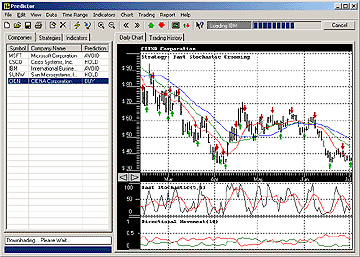# Fast Stochastic Oscillator Cross - Rising

The Fast Stochastic Oscillator Cross trading strategy is a technical analysis trading strategy that involves using the Fast Stochastic oscillator to identify potential entry and exit points for a stock. The strategy involves two main components: the Fast Stochastic oscillator and its signal line.

The oscillator is calculated by taking the difference between the current closing price and the lowest price over the specified period, divided by the difference between the highest price and the lowest price over the specified period. The resulting value is then multiplied by 100 to produce a percentage value between 0 and 100.

The signal line is a moving average of the oscillator, typically a 3-period moving average.

The trading strategy involves waiting for the Fast Stochastic oscillator to cross above or below its signal line. A cross above the signal line is considered a buy signal, while a cross below the signal line is considered a sell signal.

This strategy is based on crossings in Fast Stochastic Oscillator. Typically a buy is generated when a %K (black) line is crossing a %D (red)  line in an upward direction. When %K is crossing %D in a downward direction it is a sell signal.

This strategy is generating a buy signal on an upward crossing of %D by %K and only if that crossing occurs below a certain level a. A sell signal is generated when %D is crossed by %K in a downward direction above a certain level b. Typically a = 20 and b = 80.

### Formula

IF STO%K < a AND STO%D < a
AND STO%D >= STO%K
THEN GO LONG
ELSE
IF STO%K > b AND STO%D > b
AND STO%D <= STO%K
THEN GO SHORT
where STO%K is %K fast stochastic oscillator;
where STO%D is %D fast stochastic oscillator;
and a < b; e.g. a = 20 and b = 80

ATVI ABC AVY WMT IRM NUE BLK HNI HI TRN SNA CHE

< Back to Stock Predictor SiteOrder >